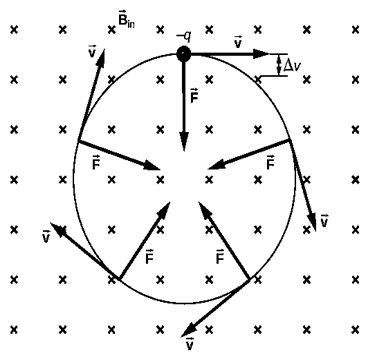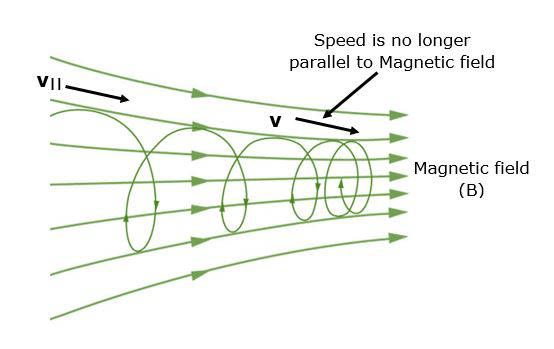# Motion of a Charged Particle in a Magnetic Field

• Last Updated : 30 Jun, 2021

This has been already learned about the interaction of electric and magnetic fields, as well as the motion of charged particles in the presence of both electric and magnetic fields. We have also deduced the relationship of the force acting on the charged particle, which is given by the Lorentz force in this instance. We’ve also learned about the magnetic force acting on a current-carrying conducting rod when it’s exposed to a magnetic field.

What happens, though, when a charged particle travels in the presence of a magnetic field? How can we define such a particle’s trajectory? This will be covered in detail in this article.

When a force acts on a particle, it is said to produce work if a component of the force is directed in the particle’s direction of motion. The magnetic force acts perpendicular to the particle’s motion in the situation under discussion when we have a charged particle carrying a charge q traveling in a uniform magnetic field of size B. In this case, we claim that the magnetic force does no work on the particle and hence no change in the particle’s velocity can be seen. When the particle’s velocity v is perpendicular to the direction of the magnetic field, we may write,

F = q (v × B)

In this case, the magnetic force is directed towards the object’s center of circular motion and functions as a centripetal force. As a result, if v and B are perpendiculars, the particle describes a circle. In other situations, if a component of velocity exists in the direction of the magnetic field B, its magnitude remains constant during the motion, because it is unaffected by a magnetic field. Furthermore, as previously mentioned, the motion caused by the perpendicular component of the velocity is circular in nature.

### Circular Motion of a Charged particle in a Magnetic Field

When a charged particle moves through a magnetic field, it experiences a force. What happens if this field is uniform across the charged particle’s motion? Which route does the particle take? In this section, we will look at the circular motion of a charged particle as well as other motions that occur when a charged particle enters a magnetic field.

The simplest instance is when a charged particle moves perpendicular to a uniform, as seen in the picture below. If the field is in a vacuum, the magnetic field is the deciding factor of motion. A charged particle in a magnetic field travels a curved route because the magnetic force is perpendicular to the direction of motion.In a region where the magnetic field is perpendicular to the paper, a negatively charged particle travels in the plane of the paper.

This curving path is followed by the particle until it forms a full circle. Another way to think about it is that the magnetic force is always perpendicular to the velocity, thus it does no work on the charged particle. As a result, the particle’s kinetic energy and speed stay constant. The speed is unaffected, but the direction is.

### Helical Motion

When the velocity vector is not perpendicular to the magnetic field vector, helical motion occurs.

The motion generated when one component of the velocity is constant in amplitude and direction (i.e., straight-line motion) and the other component is constant in speed but changes in direction evenly (i.e., circular motion) is called the Helical motion. It is the result of the combination of straight-line and circular motion.

When a charged particle travels perpendicular to a uniform B-field, as illustrated in, the simplest situation occurs. (If this happens in a vacuum, the magnetic field is the most important element influencing motion.) The magnetic force (Lorentz force) provides the centripetal force in this case, as

Fc = mv2 / r

Since, here sin θ = 1

Then, the magnetic force:

F = qvB

Now, if the Lorentz magnetic force provides the centripetal force, therefore, these force must be equal as:

qvB = mv2 / r

Solve the above expression for r as,

r = mv / qB

The radius of curvature of the path of a charged particle with mass m and charge q traveling at a speed v perpendicular to a magnetic field of strength B is denoted by r, also known as the gyroradius or cyclotron radius. In other words, it is the radius of a charged particle’s circular motion in the presence of a homogeneous magnetic field.

If the velocity is not normal to the magnetic field, then v is the perpendicular component of the velocity. Because the magnetic force is 0 for motion parallel to the field, the component of velocity parallel to the field is unaffected. In a subsequent section on spiral motion, we’ll look at the implications of this situation.

A Cyclotron resonance occurs when a particle experiences circular motion as a result of a homogeneous magnetic field. The word is derived from the name of a cyclic particle accelerator known as a cyclotron, which was demonstrated in.

The cyclotron frequency is defined as the number of cycles a particle finishes itself around a circular circuit per second and may be calculated by solving for v above and substituting in the circulation frequency such that

f = v / 2πr

or

f = qB / 2πm

Hence, The cyclotron frequency is easily expressed in radians per second as:

ω = qB / m

### Magnetic Mirror

A magnetic field arrangement in which the field intensity varies along a field line. The mirror effect causes charged particles to bounce back from the high field area, such a phenomenon is called the Magnetic mirror.Magnetic mirror and Helical Motion

If v denotes the particle’s rotational frequency. Therefore, the time span for one revolution may be expressed as follows:

Time for one revolution, T = 2π / ω = 1 / v

The pitch of a particle is the distance it moves along the direction of the magnetic field in one rotation. then:

Pitch, p = v|| T = 2πmv|| / qB

where v|| is the velocity parallel to the magnetic field.

### Sample Problems

Problem 1: Describe how a charged particle would move in a cyclotron if the frequency of the radio frequency (rf) field was doubled.

Solution:

The resonance requirement is violated and the time period of the radio frequency (rf) field is half when the frequency of the radio frequency (rf) field is doubled. As a result, radio frequency completes the cycle in the time it takes a particle to complete half a rotation inside the D’s.

Problem 2: Out of protons, neutrons, and electrons which particle may have the lowest frequency of revolution when propelled with the very same velocity normal to the magnetic field?

Solution:

When a charged particle with mass m and charge q is projected in a magnetic field B then it starts revolving with a frequency of,

f = Bq / 2πm

As a result, a high q/m ratio indicates a higher frequency, and an electron has the highest q/m ratio of the three due to its low mass. The electron’s frequency will be the highest.

Problem 3: When a proton travels in a uniform magnetic field, its velocity changes but its kinetic energy does not. Why?

Solution:

The magnetic force will be perpendicular to the direction of the proton’s travel. We know that when the force acting is perpendicular to the direction of the moving charge, the work done is zero. It indicates that kinetic energy remains constant. The force can alter the direction (velocity) of a proton but not its speed (magnitude). As a result, momentum and velocity shift.

Problem 4: Can a magnetic field accelerate a charged particle? Is it possible to enhance its speed?

Solution:

The magnetic field accelerates the charged particle by altering its velocity direction. The charged particle’s speed is unaffected by the magnetic field. The magnetic field has no effect on speed since it exerts a force perpendicular to the motion. As a result, the force cannot accomplish work on the particle. As a result, the particle’s kinetic energy cannot be changed. Therefore, it is unable to adjust the speed.

Problem 5: What is Lorentz Force? Explain.

Solution:

Lorentz force, the force exerted on a charged particle q travelling with velocity v through an electric and magnetic field E and B. The Lorentz force (named after the Dutch scientist Hendrik A. Lorentz) is the total electromagnetic force F on the charged particle, and it is given by,

F = qE + qv × B

The electric field contributes the first term. The magnetic force, which has a direction perpendicular to both the velocity and the magnetic field, is the second term. Magnetic force is proportional to q as well as the size of the vector cross product v × B. The amount of the force equals qvB sin in terms of the angle between v and B.

The velocity of a charged particle in a uniform magnetic field is an intriguing consequence of the Lorentz force. If v is perpendicular to B (i.e., there is a 90° angle between v and B), the particle will follow a circular trajectory with radius r = mv/qB. The particle orbit will be a helix with an axis parallel to the field lines if the angle is less than 90°.

If ϕ is 0, there will be no magnetic force acting on the particle, which will continue to travel along the field lines undeflected. Particle accelerators using charged particles, such as cyclotrons, take use of the fact that particles move in a circular orbit when v and B are at right angles.

My Personal Notes arrow_drop_up